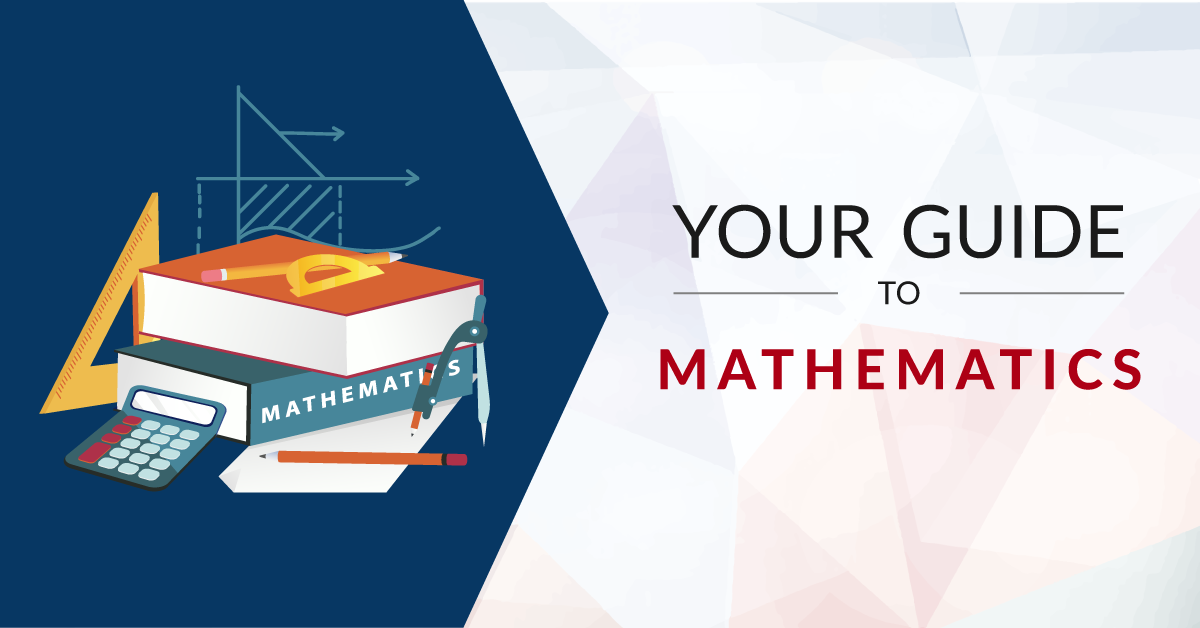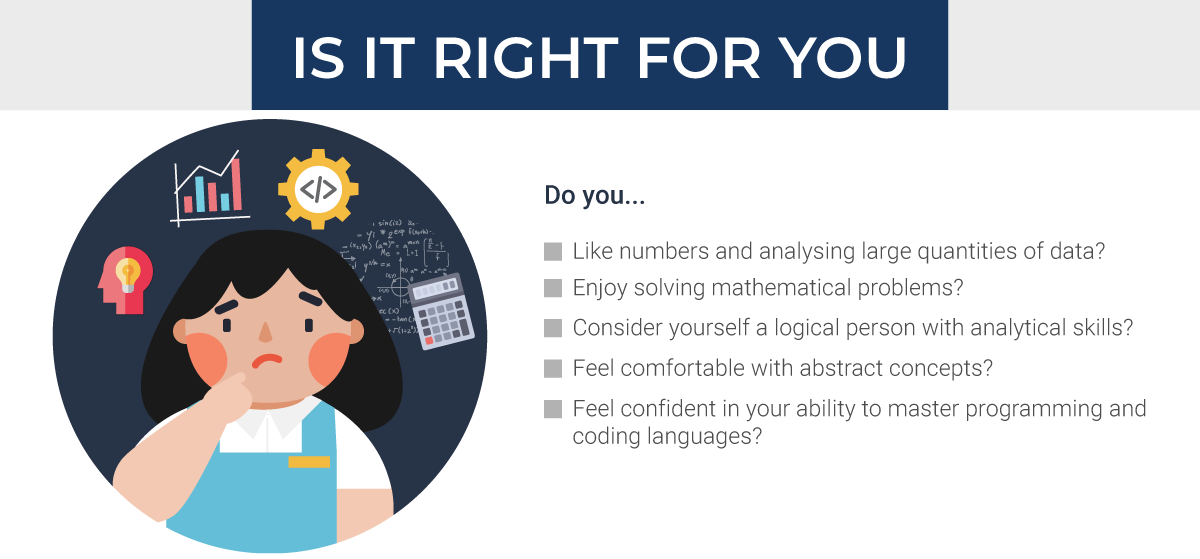# The Complete Guide to Studying Mathematics in Malaysia

What do you learn in a mathematics course and what jobs can you get with it? Access entry requirements, degree pathway and best institutions in this guide.Do you enjoy working with numbers and solving mathematical problems? Are you fascinated by the concepts and theories around mathematics? Or perhaps you’re interested in learning how to apply mathematics to everyday problems, be it in relation to data, finance or computer science.

If so, a mathematics degree may be the right match for you.

This comprehensive guide will help you find out everything there is to know about studying for a mathematics degree in Malaysia. You’ll learn about the various types of mathematics degrees, the subjects you’ll cover, what careers you can pursue as a mathematics graduate and the best universities for a mathematics degree.University of Nottingham Malaysia

Mathematics and Data Science BSc (Hons)

Ranked 22nd in the UK for Mathematics in the Subject League Table 2023, The Complete University Guide

## #1. What is Mathematics?Mathematics is the study of numbers, formulas, structures and spaces. As a field of study, mathematics often involves the study of theoretical concepts and practical applications to solve real-world problems.

Whether we like it or not, we live in a world dominated by numbers, equations and algorithms. Furthermore, in this age of data science, where massive data sets can only be understood through statistical models and analysis, the importance of mathematics cannot be denied. In fact, mathematical principles play a key role in the research and innovation of science, engineering, technology and business.

As part of your mathematical studies, you will be exploring mathematical concepts and theories and learning how to apply them to an array of large, complex problems. Depending on what type of mathematics degree you take, you’ll not only cover the fundamentals of mathematics (calculus, algebra, statistics) but also specialised modules in computing, data science, finance and business.

### Fields of Mathematics Degrees in Malaysia

The scope of a mathematics degree varies by university. Some universities offer mathematics as a study on its own (i.e. single-subject degree), while others offer the study of mathematics in conjunction with other related subjects.

Common types of mathematics degrees are pure mathematics, applied mathematics, statistics as well as mathematics offered together with computer science, finance and business.

Field What It Is
Applied Mathematics The application of mathematics to various fields such as physics, engineering and computer science
Mathematics and Business Integrates mathematics with elements of business, finance and economics
Mathematics and Computer Science Covers the foundation of mathematics and the essentials of computer science to develop programs and software
Mathematics and Data Science Combines mathematics with applications to the field of data science covering machine learning, artificial intelligence and statistical modelling
Mathematics and Finance Primarily concerned with financial mathematics, statistics and probability
Pure Mathematics The study of mathematical concepts and structures that underlie all mathematics
Statistics The science that relates to collecting, analysing and interpreting data

## #2. Entry Requirements for Mathematics

### a) What are the subjects required to study Mathematics?

In general, you’ll need to have studied the following subjects at SPM level or equivalent:

• Mathematics

As for A Levels, STPM or equivalent, you will need to take the following subjects to study for a Degree in Mathematics:

• Mathematics

### (b) Mathematics Degree requirements

To pursue a Degree in Mathematics, you need to complete a pre-university programme and meet the entry requirements:

Important Note: Entry requirements for some universities may be higher, so it’s important that you scrutinise the details carefully.

## #3. Studying Mathematics in Malaysia

### a) What subjects will you study in Mathematics?

The modules you cover in a mathematics programme are designed to expose you to a combination of theory and abstract mathematics as well as how you can practically apply it to the real world. During the course of your study, you’ll develop the ability to think critically and analytically to analyse situations, organise information and devise solutions to complex problems.

In most mathematics degrees, you’ll take introductory classes in your first year that will introduce you to basic mathematical concepts and components. As you progress in your studies, you’ll cover more complex and focused topics that’ll strengthen your knowledge of the field. This will depend whether your mathematics degree is a single-subject degree (i.e. mathematics only) or a degree where mathematics is studied with other related subjects (e.g. finance, computer science, business, management, data science, etc.).

Here are some core modules that you may cover as the foundation to your mathematical studies:

• Calculus
• Algebra
• Probability
• Statistics
• Programming and algorithms

For mathematics degrees with other subject fields, some of your modules may include:

• Mathematics and Computer Science: Operating systems, data structures, advanced programming, applied statistical modelling
• Mathematics and Data Science: Machine learning, artificial intelligence, statistical modelling
• Mathematics and Finance: Financial derivatives, financial modelling, financial economics

As part of your mathematics degree, you may be required to complete an internship and/or a final year project.

### b) How long is a Degree in Mathematics?

A Degree in Mathematics typically takes about 3 years to complete.

### c) How much does it cost to study Mathematics in Malaysia?

A Mathematics degree could range from RM34,100 – RM115,700.University of Nottingham Malaysia

Foundation in Engineering

Interact with the best lecturers from the Faculty of Engineering, consistently ranked in the top 20 in the UK, The Complete University Guide

## #4. Your Education Pathway for MathematicsUpon the completion of your SPM or equivalent qualification, you can opt to enrol into a pre-university (STPM, A Levels, etc.) or Foundation in Science programme.

Completing a pre-university or a foundation programme will enable you to proceed to a Degree in Mathematics.

## #5. Should You Study Mathematics?

###a) Is Mathematics right for you?

If you’re wondering whether you should pursue this programme, here are some questions to think about:

• Are you good with numbers and analysing large quantities of data?
• Do you enjoy solving mathematical problems?
• Do you consider yourself a logical person with analytical skills?
• Are you comfortable with abstract concepts?
• Are you confident in your ability to master programming and coding languages?
• Will you be comfortable using mathematical software to analyse data, solve problems and create models?

If you answered yes to most of these questions, this analytical course may be right for you!

### b) Skills required to pursue Mathematics

Here are some key skills an excellent Mathematics graduate should have:

• High numeracy and mathematical ability
• Computer literacy, including knowledge of programming languages, coding and data analysis tools
• Good analytical skills
• Attention to detail and high level of accuracy
• Ability to interpret mathematical results in real-world terms
• Good problem-solving skills
• Ability to work both independently as well as with teams
• Ability to communicate findings clearly and effectivelyUniversity of Nottingham Malaysia

Mathematics and Data Science BSc (Hons)

Ranked 22nd in the UK for Mathematics in the Subject League Table 2023, The Complete University Guide

## #6. Jobs You Can Get with a Mathematics Degree

Mathematics graduates are well-equipped for careers that require logical, analytical or strategic thinking. With a mathematics degree, you can go into finance, computing, management, research and academia. This includes working in financial institutions, IT companies, market research companies, government agencies and universities.

Here are some careers you can pursue with a Mathematics qualification:

• Mathematician
• Statistician
• Operations Research Analyst
• Data Scientist
• Investment Analyst
• Quantitative Analyst
• Software Engineer
• Cryptologist
• Research Analyst
• Lecturer

## #7. Best Institutions for Mathematics in Malaysia

If mathematics is what you’re passionate about, then check out some of the best institutions for Mathematics in Malaysia.